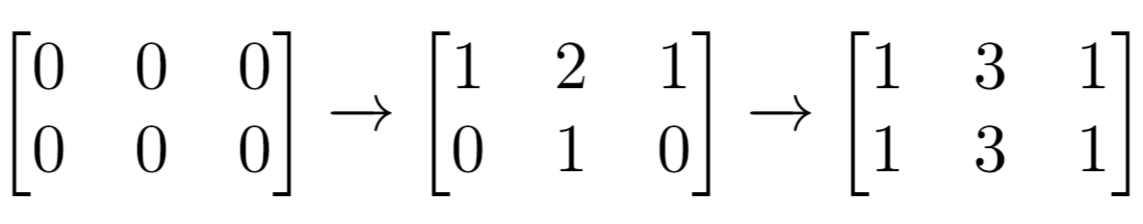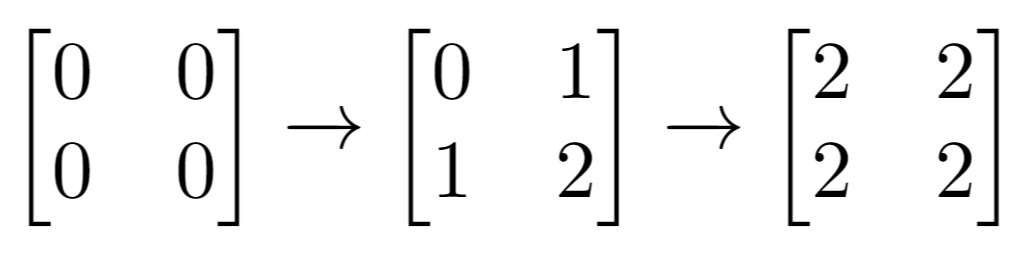## Algorithm

Problem Name: 1252. Cells with Odd Values in a Matrix

There is an `m x n` matrix that is initialized to all `0`'s. There is also a 2D array `indices` where each `indices[i] = [ri, ci]` represents a 0-indexed location to perform some increment operations on the matrix.

For each location `indices[i]`, do both of the following:

1. Increment all the cells on row `ri`.
2. Increment all the cells on column `ci`.

Given `m`, `n`, and `indices`, return the number of odd-valued cells in the matrix after applying the increment to all locations in `indices`.

Example 1:```Input: m = 2, n = 3, indices = [[0,1],[1,1]]
Output: 6
Explanation: Initial matrix = [[0,0,0],[0,0,0]].
After applying first increment it becomes [[1,2,1],[0,1,0]].
The final matrix is [[1,3,1],[1,3,1]], which contains 6 odd numbers.
```

Example 2:```Input: m = 2, n = 2, indices = [[1,1],[0,0]]
Output: 0
Explanation: Final matrix = [[2,2],[2,2]]. There are no odd numbers in the final matrix.
```

Constraints:

• `1 <= m, n <= 50`
• `1 <= indices.length <= 100`
• `0 <= ri < m`
• `0 <= ci < n`

## Code Examples

### #1 Code Example with Javascript Programming

```Code - Javascript Programming```

``````
const oddCells = function (n, m, indices) {
const oddRows = new BitSet(n),
oddCols = new BitSet(m)
let cntRow = 0,
cntCol = 0
for (let idx of indices) {
oddRows.flip(idx)
oddCols.flip(idx)
cntRow += oddRows.get(idx) ? 1 : -1
cntCol += oddCols.get(idx) ? 1 : -1
}
return (m - cntCol) * cntRow + (n - cntRow) * cntCol
}

class BitSet {
constructor(n) {
this.arr = Array(n).fill(0)
}
flip(idx) {
this.arr[idx] = this.arr[idx] === 0 ? 1 : 0
}
get(idx) {
return this.arr[idx]
}
}
``````
Copy The Code &

Input

cmd
m = 2, n = 3, indices = [[0,1],[1,1]]

Output

cmd
6

### #2 Code Example with Python Programming

```Code - Python Programming```

``````
from collections import Counter as cnt

class Solution:
def oddCells(self, n: int, m: int, indices: List[List[int]]) -> int:
row, col = cnt(r for r, c in indices), cnt(c for r, c in indices)
return sum((row[i] + col[j]) % 2 for i in range(n) for j in range(m))
``````
Copy The Code &

Input

cmd
m = 2, n = 3, indices = [[0,1],[1,1]]

Output

cmd
6

### #3 Code Example with C# Programming

```Code - C# Programming```

``````
namespace LeetCode
{
public class _1252_CellsWithOddValuesInAMatrix
{
public int OddCells(int n, int m, int[][] indices)
{
var row = new bool[n];
var col = new bool[m];

foreach (var indice in indices)
{
row[indice] = !row[indice];
col[indice] = !col[indice];
}

var count = 0;
for (int i = 0; i < n; i++)
for (int j = 0; j < m; j++)
if (row[i] != col[j]) count++;

return count;
}
}
}
``````
Copy The Code &

Input

cmd
m = 2, n = 3, indices = [[0,1],[1,1]]

Output

cmd
6